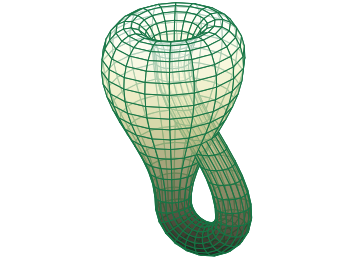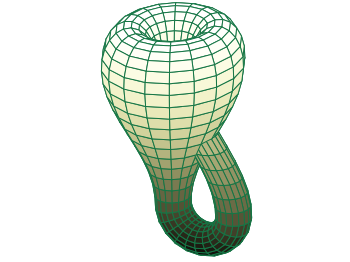# Gnuplotting

Create scientific plots using gnuplot

June 7th, 2011 | 6 Comments

A Klein bottle is a non-orientable surface, which has no defined left and right, as stated on Wikipedia. There we can also find a Gnuplot plot of the bottle, which we want to fine-tune a little bit in order to reach the result in Fig. 1.Fig. 1 Klein bottle (code to produce this figure)

In order to reach Fig. 1 we start with the definition of the parametric functions given in Wikipedia and do a simple `pm3d` plot with them.

```set parametric
x(u,v)= v<2*pi ? (2.5-1.5*cos(v))*cos(u) : \
v<3*pi ? -2+(2+cos(u))*cos(v)    : \
-2+2*cos(v)-cos(u)
y(u,v)= v<2*pi ? (2.5-1.5*cos(v))*sin(u) : \
sin(u)
z(u,v)= v<pi   ? -2.5*sin(v)             : \
v<2*pi ? 3*v-3*pi                : \
v<3*pi ? (2+cos(u))*sin(v)+3*pi  : \
-3*v+12*pi
splot x(u,v),y(u,v),-z(u,v) w pm3d
```

The result is shown in Fig. 2.Fig. 2 Klein bottle plotted only with pm3d (code to produce this figure)

Now we add some lines to the surface and hide parts, which are not visible in 3d.

```set pm3d depthorder hidden3d 1
set hidden3d
```

Here the `depthorder` option takes care of the right positioning of the bottleneck going back into the bottle, which is not correct in Fig. 2. The `hidden3d 1` option draws lines in the right order for a correctly looking 3d plot using line style 1. The additional `set hidden3d` command takes care of showing only those lines that are visible in 3d. These options will result in Fig. 3.Fig. 3 Klein bottle plotted only with pm3d and hidden3d (code to produce this figure)

In order to reach at Fig. 1 we just have to set the surface to be transparent, which can be done by the `set style fill` command.

```set style fill transparent solid 0.65
```

5.John says: